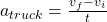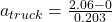A(n) 1400-kg car going at 6.32 m/s in the positive x direction collides with a 2900-kg truck at rest. The collision is totally inelastic and

Question

A(n) 1400-kg car going at 6.32 m/s in the positive x direction collides with a 2900-kg truck at rest. The collision is totally inelastic and takes place over an interval of 0.203 s. Assume that no brakes are applied during the collision and the car strikes the rear of the truck. Neglect the friction between the vehicles and the ground.
What is the average x component of the acceleration of the car during the collision?
What is the average x component of the acceleration of the truck during the collision?

in progress 0
6 months 2021-08-10T01:58:00+00:00 1 Answers 0 views 0

a) Acceleration of the car is given as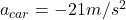b) Acceleration of the truck is given as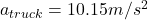Explanation:

As we know that there is no external force in the direction of motion of truck and car

So here we can say that the momentum of the system before and after collision must be conserved

So here we will have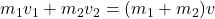now we have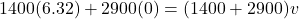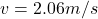a) For acceleration of car we know that it is rate of change in velocity of car

so we have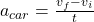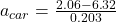b) For acceleration of truck we will find the rate of change in velocity of the truck

so we have